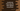# JavaScript program to find the most frequent element in an Array## Introduction:

In this tutorial, we will learn how to find the most frequent element or element with the highest occurrence in an array in `JavaScript`. I will show you three different ways to solve this problem.

### Problem statement:

You need to write one program in `JavaScript` to find out the element with the highest occurrence in that array. We will take one integer array with all examples.

For example, if the array is:

``[1, 2, 3, 4, 1, 1, 2, 3];``

The frequency of the numbers are:

Number Frequency
1 3
2 2
3 2
4 1

The program will print 1, as three 1 are there in this array and it is the highest frequency.

### Method 1: By using a loop and a dictionary:

The following example uses a `for` loop and a `dictionary` to solve this problem. It adds the frequency of the numbers to a dictionary to find the element with highest occurrence in the array:

``````// 1
const givenArray = [1, 2, 3, 4, 1, 1, 2, 3, 4];

// 2
let itemsMap = {};
let maxValue = 0;
let maxCount = 0;

// 3
for (let item of givenArray) {
// 4
if (itemsMap[item] == null) {
itemsMap[item] = 1;
} else {
itemsMap[item]++;
}

//5
if (itemsMap[item] > maxCount) {
maxValue = item;
maxCount = itemsMap[item];
}
}

// 6
console.log(`Value : \${maxValue}, Count : \${maxCount}`);``````

Get it on GitHub

#### Explanation:

The commented numbers in the above example denote the step numbers below:

1. The array is assigned to the variable `givenArray`. We are finding the element with the highest occurrence in this array. The `maxValue` and `maxCount` variables are initialized as 0 to assign the highest occurring element and the total frequency of that element.

2. The `itemsMap` is an empty dictionary. This dictionary will be used to hold the number and its occurrence count as key-value pairs.

3. It uses a `for` loop to iterate over the array elements.

4. For each element, it checks if the current element is an existing `key` of the dictionary or not. If not, it adds this element as `key` and assigns 1 as its `value`. Else, it increments the `value` for that `key` by 1.

5. If the `value` for the current number is greater than `maxCount`, assign this element to `maxValue` and assign its count to `maxCount`.

It will print the below output:

``Value : 1, Count : 3``

### Method 2: By using the `reduce` and `filter` methods:

We can use the `reduce` and `filter` methods to find the most frequent element of an array as shown in the following example:

``````const givenArray = [1, 2, 3, 4, 1, 1, 2, 3, 4];

const maxValue = givenArray.reduce((previous, current, _, arr) => {
if (
arr.filter((item) => item === previous).length >
arr.filter((item) => item === current).length
) {
return previous;
} else {
return current;
}
});

console.log(`Element with highest frequency: \${maxValue}`);``````

Get it on GitHub

• The `reduce()` method takes one reducer callback function as its parameter and it executes it on all the array elements. The reducer function provides the `previous` and the `current` element. It uses the `filter` method to compare the number of occurrences of the `previous` and `current` elements. It returns the element which has more frequency in the array. It will return the element with highest occurrence eventually.

You can also write the above program like below:

``````const givenArray = [1, 2, 3, 4, 1, 1, 2, 3, 4];

const maxValue = givenArray.reduce((previous, current, i, arr) =>
arr.filter((item) => item === previous).length >
arr.filter((item) => item === current).length
? previous
: current
);

console.log(`Maximum occurrence value: \${maxValue}`);``````

### Method 3: By using the `sort` method:

The `sort()` method sorts an array. We can also pass one function to the `sort` method to define the sorting behavior. The following example shows how to get the highest frequency element of an array with the `sort` method:

``````const givenArray = [1, 2, 3, 4, 1, 1, 2, 3, 4];

const maxValue = givenArray
.sort(
(previous, current) =>
givenArray.filter((item) => item === previous).length -
givenArray.filter((item) => item === current).length
)
.pop();

console.log(`Maximum occurrence value: \${maxValue}`);``````

Get it on GitHub

The `sort` method overwrites the original array. In the above example, it will push the elements with the highest occurrence to the end. We are using the `pop()` method to get the last element of the sorted array i.e. it will return the element with the maximum occurrences.